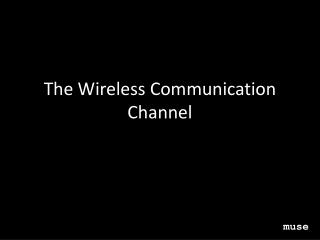DownloadDownload PresentationThe Wireless Communication Channel

# The Wireless Communication Channel

Télécharger la présentation## The Wireless Communication Channel

- - - - - - - - - - - - - - - - - - - - - - - - - - - E N D - - - - - - - - - - - - - - - - - - - - - - - - - - -
##### Presentation Transcript

1. Objectives • Understand fundamentals associated with free-space propagation. • Define key sources of propagation effects both at the large- and small-scales • Understand the key differences between a channel for a mobile communications application and one for a wireless sensor network muse

2. Objectives (cont.) • Define basic diversity schemes to mitigate small-scale effects • Synthesize these concepts to develop a link budget for a wireless sensor application which includes appropriate margins for large- and small-scale propagation effects muse

3. Outline • Free-space propagation • Large-scale effects and models • Small-scale effects and models • Mobile communication channels vs. wireless sensor network channels • Diversity schemes • Link budgets • Example Application: WSSW

4. Free-space propagation • Scenario Free-space propagation: 1 of 4

5. Relevant Equations • Friis Equation • EIRP Free-space propagation: 2 of 4

6. Alternative Representations • PFD • Friis Equation in dBm Free-space propagation: 3 of 4

7. Issues • How useful is the free-space scenario for most wireless systems? Free-space propagation: 4 of 4

8. Outline • Free-space propagation • Large-scale effects and models • Small-scale effects and models • Mobile communication channels vs. wireless sensor network channels • Diversity schemes • Link budgets • Example Application: WSSW

9. Large-scale effects • Reflection • Diffraction • Scattering Large-scale effects: 1 of 7

10. Modeling Impact of Reflection • Plane-Earth model Large-scale effects: 2 of 7 Fig. Rappaport

11. Modeling Impact of Diffraction • Knife-edge model Large-scale effects: 3 of 7 Fig. Rappaport

12. Modeling Impact of Scattering • Radar cross-section model Large-scale effects: 4 of 7

13. Modeling Overall Impact • Log-normal model • Log-normal shadowing model Large-scale effects: 5 of 7

14. Log-log plot Large-scale effects: 6 of 7

15. Issues • How useful are large-scale models when WSN links are 10-100m at best? Free-space propagation: 7 of 7 Fig. Rappaport

16. Outline • Free-space propagation • Large-scale effects and models • Small-scale effects and models • Mobile communication channels vs. wireless sensor network channels • Diversity schemes • Link budgets • Example Application: WSSW

17. Small-scale effects • Multipath • Time and frequency response • Models Small-scale effects: 1 of 14

18. Multipath • Scenario • Equations Small-scale effects: 2 of 14

19. Case 1: primary and secondary paths arrive at same time (path Δ = 0) Multipath component: -1.7 dB down Time and Frequency Response Small-scale effects: 3 of 14

20. Case 2: primary and secondary paths arrive at same time (path Δ = 1.5m) Time and Frequency Response Small-scale effects: 4 of 14

21. Case 3: primary and secondary paths arrive at same time (path Δ = 4.0m) Time and Frequency Response Small-scale effects: 5 of 14

22. Case 4: primary and secondary paths arrive at same time (path Δ = 4.5m) Time and Frequency Response Small-scale effects: 6 of 14

23. Real World Data Fig. Frolik – IEEE TWC Apr. 07 Small-scale effects: 7 of 14

24. Randomness in the Channel • Sources • Impact Small-scale effects: 8 of 14

25. Statistical Channel Models • TWDP Small-scale effects: 9 of 14

26. Baseline: Rayleigh Distribution • Scenario • Equations Small-scale effects: 10 of 14

27. Cumulative Distribution Function Small-scale effects: 11 of 14

28. Ricean: Less Severe than Rayleigh Small-scale effects: 12 of 14

29. More Severe than Rayleigh? Small-scale effects: 13 of 14

30. Importance of Proper Model Small-scale effects: 14 of 14

31. Outline • Free-space propagation • Large-scale effects and models • Small-scale effects and models • Mobile communication channels vs. wireless sensor network channels • Diversity schemes • Link budgets • Example Application: WSSW

32. Mobile WSN Mobile vs. WSN channels Mobile vs. WSN: 1 of 3

33. Mobile WSN Channel Effects Mobile vs. WSN: 2 of 3 Fig. Rappaport

34. Real world data revisited Fig. Frolik – IEEE TWC Apr. 07 Mobile vs. WSN: 3 of 3

35. Outline • Free-space propagation • Large-scale effects and models • Small-scale effects and models • Mobile communication channels vs. wireless sensor network channels • Diversity schemes • Link budgets • Example Application: WSSW

36. Diversity schemes • Time • Space • Frequency Diversity schemes: 1 of 3

37. Approaches • MRC • Selection Diversity schemes: 2 of 3

38. Benefits Fig. Bakir – IEEE TWC Diversity schemes: 3 of 3

39. Outline • Free-space propagation • Large-scale effects and models • Small-scale effects and models • Mobile communication channels vs. wireless sensor network channels • Diversity schemes • Link budgets • Example Application: WSSW

41. Antenna Requirement? Link budgets: 2 of 5

43. Path loss exponent Link budgets: 4 of 5

44. Margin Calculation Link budgets: 5 of 5

45. Outline • Free-space propagation • Large-scale effects and models • Small-scale effects and models • Mobile communication channels vs. wireless sensor network channels • Diversity schemes • Link budgets • Example Application: WSSW

46. Example: WSSW • Motivation • Approach WSSW: 1 of 2

47. WSSW Results WSSW: 2 of 2

48. Conclusions - 1 • As intuitively suspected, signal strength on average decreases with T-R distance • Large-scale effects determine the rate of signal strength degradation with distance • Small-scale effects may severely impact signal strength in highly reflective environments • Diversity schemes can mitigate the small-scale effects muse

49. Conclusions - 2 • WSN have unique constrains which may not be best modeled using mobile communication methods • Link budgets are critical in order ascertain requisite transmit powers, expected connectivity length, etc. • Sensor nodes themselves can be utilized to ascertain channel characteristics muse